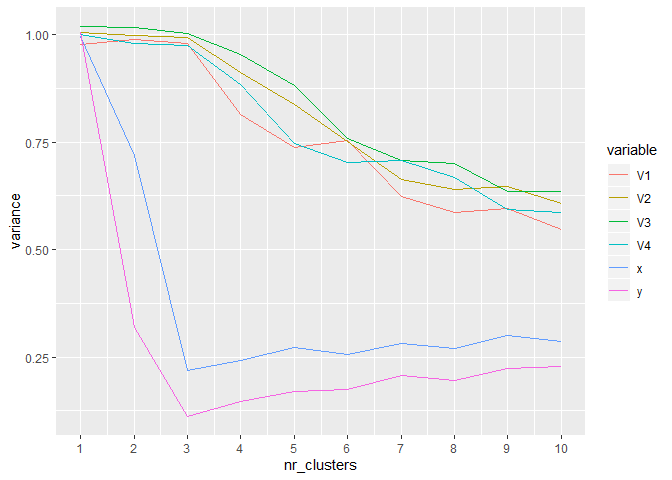Simulated data with missings

First we create a random dataset with some structure and a few uncorrelated variables

### Random Dataset
set.seed(739)
n <- 7500 # numer of points
nr_other_vars <- 4
mat <- matrix(rnorm(nr_other_vars*n),n,nr_other_vars)
me<-4 # mean
x <- c(rnorm(n/3,me/2,1),rnorm(2*n/3,-me/2,1))
y <- c(rnorm(n/3,0,1),rnorm(n/3,me,1),rnorm(n/3,-me,1))
true_clust <- c(rep(1,n/3),rep(2,n/3),rep(3,n/3)) # true clusters
dat <- cbind(mat,x,y)
dat<- as.data.frame(scale(dat)) # scaling
summary(dat)
#>        V1                 V2                  V3
#>  Min.   :-3.40352   Min.   :-4.273673   Min.   :-3.82710
#>  1st Qu.:-0.67607   1st Qu.:-0.670061   1st Qu.:-0.66962
#>  Median : 0.01295   Median :-0.006559   Median :-0.01179
#>  Mean   : 0.00000   Mean   : 0.000000   Mean   : 0.00000
#>  3rd Qu.: 0.67798   3rd Qu.: 0.684672   3rd Qu.: 0.67221
#>  Max.   : 3.35535   Max.   : 3.423416   Max.   : 3.80557
#>        V4                  x                 y
#>  Min.   :-3.652267   Min.   :-2.1994   Min.   :-2.151001
#>  1st Qu.:-0.684359   1st Qu.:-0.7738   1st Qu.:-0.975136
#>  Median : 0.001737   Median :-0.2901   Median : 0.009932
#>  Mean   : 0.000000   Mean   : 0.0000   Mean   : 0.000000
#>  3rd Qu.: 0.687404   3rd Qu.: 0.9420   3rd Qu.: 0.975788
#>  Max.   : 3.621530   Max.   : 2.8954   Max.   : 2.265420

One can clearly see the three clusters

plot(dat$x,dat$y)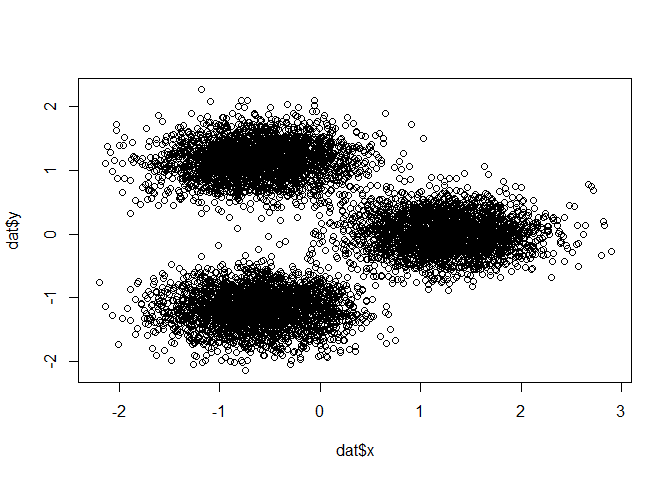We create a 20% missings using a custom function

dat_with_miss <- miss_sim(dat,p=.2,seed_nr=120)
summary(dat_with_miss)
#>        V1                V2                V3                V4
#>  Min.   :-3.4035   Min.   :-4.2737   Min.   :-3.8271   Min.   :-3.5844
#>  1st Qu.:-0.6756   1st Qu.:-0.6757   1st Qu.:-0.6634   1st Qu.:-0.6742
#>  Median : 0.0163   Median :-0.0104   Median :-0.0092   Median : 0.0194
#>  Mean   : 0.0024   Mean   :-0.0063   Mean   : 0.0027   Mean   : 0.0117
#>  3rd Qu.: 0.6886   3rd Qu.: 0.6683   3rd Qu.: 0.6774   3rd Qu.: 0.7010
#>  Max.   : 3.2431   Max.   : 3.4234   Max.   : 3.8056   Max.   : 3.6215
#>  NA's   :1513      NA's   :1499      NA's   :1470      NA's   :1486
#>        x                 y
#>  Min.   :-2.1994   Min.   :-2.1510
#>  1st Qu.:-0.7636   1st Qu.:-0.9745
#>  Median :-0.2955   Median : 0.0065
#>  Mean   : 0.0022   Mean   :-0.0019
#>  3rd Qu.: 0.9473   3rd Qu.: 0.9689
#>  Max.   : 2.8954   Max.   : 2.2654
#>  NA's   :1580      NA's   :1516
mis_ind <- is.na(dat_with_miss) # missing indicator

The correlation matrix of the missing indicator shows that the missings are correlated - thus we are not in a missing completely at random stetting:

corrplot(cor(mis_ind),method="number")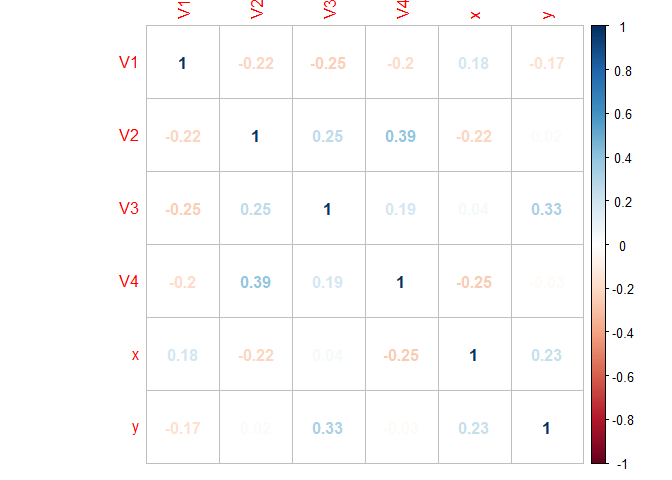Median or random imputation

Clearly, an imputation with the median value does a pretty bad job here:

dat_median_imp <- dat_with_miss
for (j in 1:dim(dat)) {
dat_median_imp[,j] <- Hmisc::impute(dat_median_imp[,j],fun=median)
}
imp <- factor(pmax(mis_ind[,5],mis_ind[,6]),labels=c("Original","Imputed")) # point is imputed if x or y is imputed
ggplot(dat_median_imp) + geom_point(aes(x=x,y=y,color=imp))
#> Don't know how to automatically pick scale for object of type impute. Defaulting to continuous.
#> Don't know how to automatically pick scale for object of type impute. Defaulting to continuous.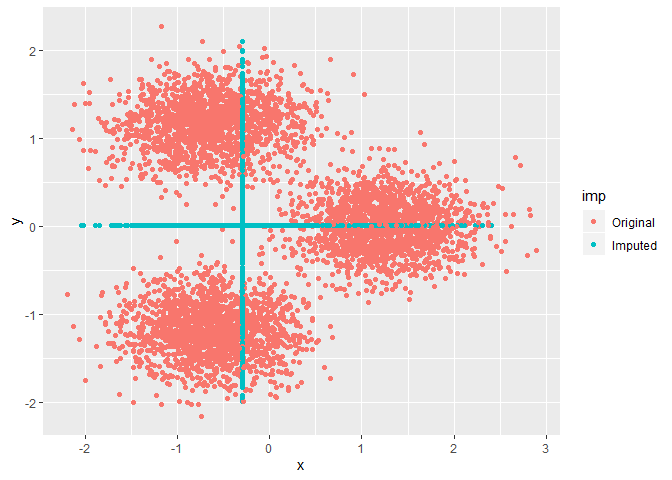But also a random imputation is not much better: it creates plenty of points in areas with no data

dat_random_imp <- dat_with_miss
for (j in 1:dim(dat)) {
dat_random_imp[,j] <- impute(dat_random_imp[,j],fun="random")
}
imp <- factor(pmax(mis_ind[,5],mis_ind[,6]),labels=c("Original","Imputed")) # point is imputed if x or y is imputed
ggplot(dat_random_imp) + geom_point(aes(x=x,y=y,color=imp))
#> Don't know how to automatically pick scale for object of type impute. Defaulting to continuous.
#> Don't know how to automatically pick scale for object of type impute. Defaulting to continuous.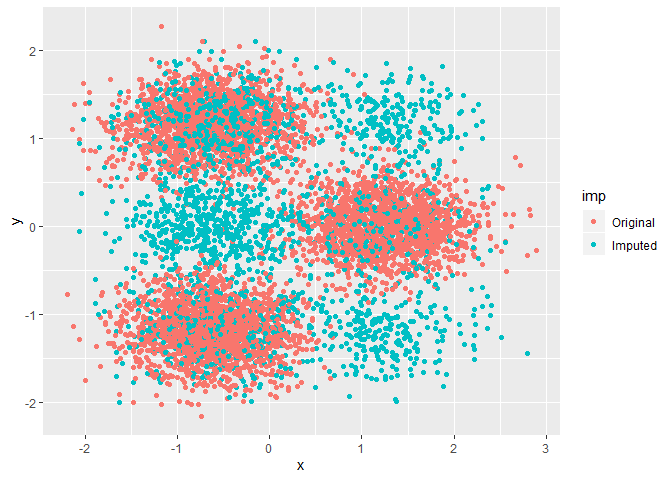A cluster base on random imputation will thus not provide good results (even if we “know” the number of clusters)

tic("Clustering based on random imputation")
cl_compare <- KMeans_arma(data=dat_random_imp,clusters=3,n_iter=100,seed=751)
toc()
#> Clustering based on random imputation: 0.03 sec elapsed
dat_random_imp$pred <- predict_KMeans(dat_random_imp,cl_compare) ggplot(dat_random_imp) + geom_point(aes(x=x,y=y,color=factor(pred))) #> Don't know how to automatically pick scale for object of type impute. Defaulting to continuous. #> Don't know how to automatically pick scale for object of type impute. Defaulting to continuous.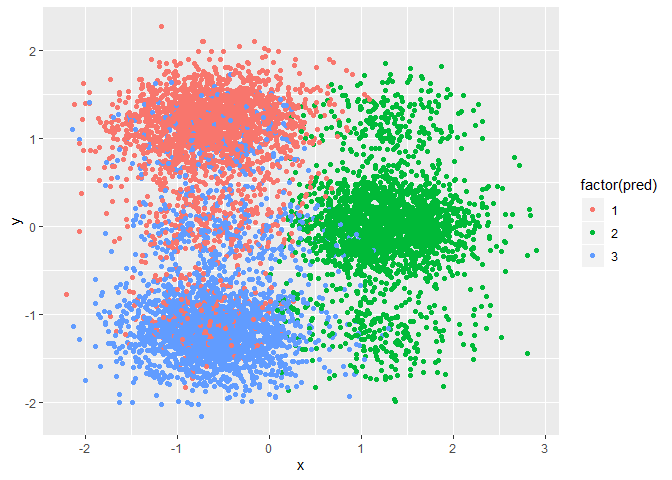Solution: ClustImpute We’ll now use ClustImpute and also measure the run-time. In short, the algorithm follows these steps 1. It replaces all NAs by random imputation, i.e., for each variable with missings, it draws from the marginal distribution of this variable not taking into account any correlations with other variables 2. Weights <1 are used to adjust the scale of an observation that was generated in step 1. The weights are calculated by a (linear) weight function that starts near zero and converges to 1 at n_end. 3. A k-means clustering is performed with a number of c_steps steps starting with a random initialization. 4. The values from step 2 are replaced by new draws conditionally on the assign cluster from step 3. 5. Steps 2-4 are repeated nr_iter times in total. The k-means clustering in step 3 uses the previous cluster centroids for initialization. 6. After the last draws a final k-means clustering is performed. The intuition is that observation should be clustered with other observations mainly based on their observed values, while the resulting clusters provide donors for the missing value imputation, so that subsequently all variables can be used for the clustering. nr_iter <- 10 # iterations of procedure n_end <- 10 # step until convergence of weight function to 1 nr_cluster <- 3 # number of clusters c_steps <- 50 # numer of cluster steps per iteration tic("Run ClustImpute") res <- ClustImpute(dat_with_miss,nr_cluster=nr_cluster, nr_iter=nr_iter, c_steps=c_steps, n_end=n_end) toc() #> Run ClustImpute: 0.41 sec elapsed ClustImpute provides several results: str(res) #> List of 5 #>$ complete_data  :'data.frame': 7500 obs. of  6 variables:
#>   ..$V1: num [1:7500] 1.403 -0.309 -0.214 -1.286 -0.202 ... #> ..$ V2: num [1:7500] -1.4579 -0.7899 -0.9775 -0.1607 -0.0413 ...
#>   ..$V3: num [1:7500] 0.7836 -0.3234 0.686 -0.0461 0.3609 ... #> ..$ V4: num [1:7500] 0.604 -0.251 -0.122 -1.287 0.807 ...
#>   ..$x : num [1:7500] 2.568 1.203 0.633 1.146 1.647 ... #> ..$ y : num [1:7500] -0.5077 -0.2453 0.022 0.0522 -0.0454 ...
#>  $clusters : int [1:7500] 2 2 2 2 2 2 2 2 2 2 ... #>$ centroids      : num [1:3, 1:6] 0.0879 -0.0638 -0.0568 0.108 -0.0138 ...
#>  $imp_values_mean: num [1:11, 1:7] 0.02068 0.01276 0.00702 -0.00798 -0.01743 ... #> ..- attr(*, "dimnames")=List of 2 #> .. ..$ : chr [1:11] "mean_imp" "" "" "" ...
#>   .. ..$: chr [1:7] "V1" "V2" "V3" "V4" ... #>$ imp_values_sd  : num [1:11, 1:7] 0.987 0.999 1.016 0.978 0.986 ...
#>   ..- attr(*, "dimnames")=List of 2
#>   .. ..$: chr [1:11] "sd_imp" "" "" "" ... #> .. ..$ : chr [1:7] "V1" "V2" "V3" "V4" ...
#>  - attr(*, "class")= chr "kmeans_ClustImpute"
#>  - attr(*, "nr_iter")= num 10
#>  - attr(*, "c_steps")= num 50
#>  - attr(*, "wf")=function (n, n_end = 10)
#>  - attr(*, "n_end")= num 10
#>  - attr(*, "seed_nr")= num 150519

We’ll first look at the complete data and clustering results. Quite obviously, it gives better results then median / random imputation.

ggplot(res$complete_data,aes(x,y,color=factor(res$clusters))) + geom_point()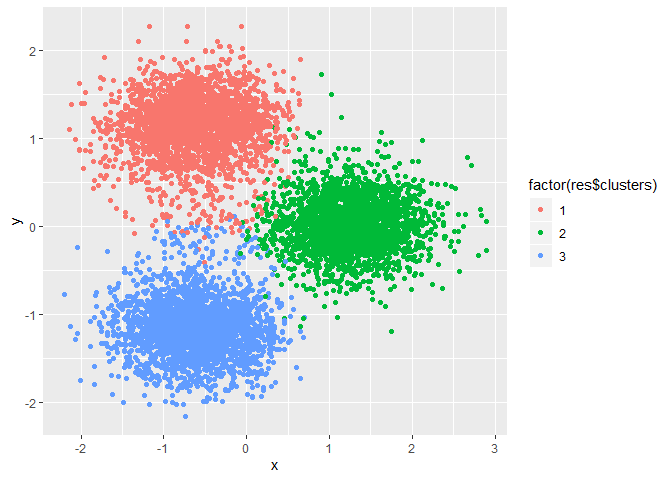Packages like MICE compute a traceplot of mean and variance for various chain. Here we only have a single realization and thus re-run ClustImpute with various seeds to obtain different realizations.

res2 <- ClustImpute(dat_with_miss,nr_cluster=nr_cluster, nr_iter=nr_iter, c_steps=c_steps, n_end=n_end,seed_nr = 2)
res3 <- ClustImpute(dat_with_miss,nr_cluster=nr_cluster, nr_iter=nr_iter, c_steps=c_steps, n_end=n_end,seed_nr = 3)
mean_all <- rbind(res$imp_values_mean,res2$imp_values_mean,res3$imp_values_mean) sd_all <- rbind(res$imp_values_sd,res2$imp_values_sd,res3$imp_values_sd)
mean_all <- cbind(mean_all,seed=rep(c(150519,2,3),each=11))
sd_all <- cbind(sd_all,seed=rep(c(150519,2,3),each=11))

The realizations mix nicely with each other.

ggplot(as.data.frame(mean_all)) + geom_line(aes(x=iter,y=V1,color=factor(seed))) + ggtitle("Mean")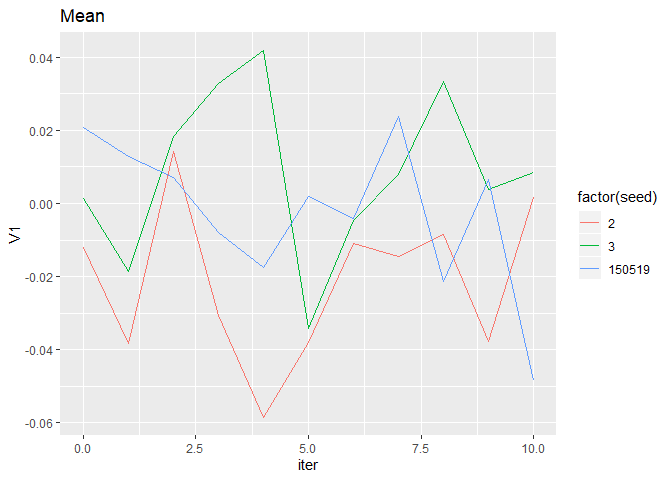ggplot(as.data.frame(sd_all)) + geom_line(aes(x=iter,y=V1,color=factor(seed))) + ggtitle("Std. dev.")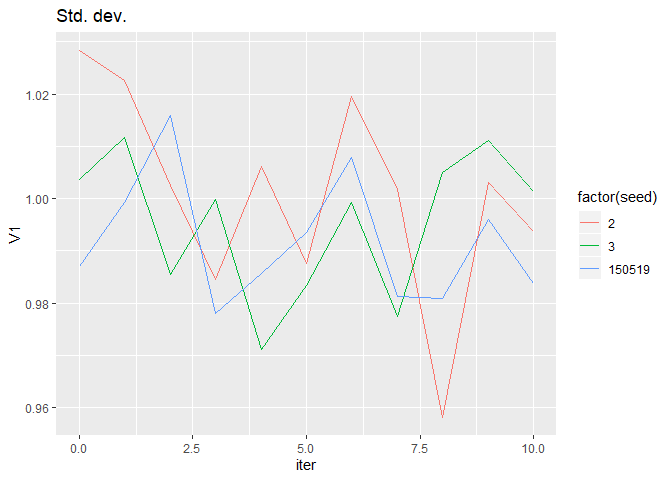Quality of imputation and cluster results

Marginal distributions

We compare marginal distributions using a violin plot of x and y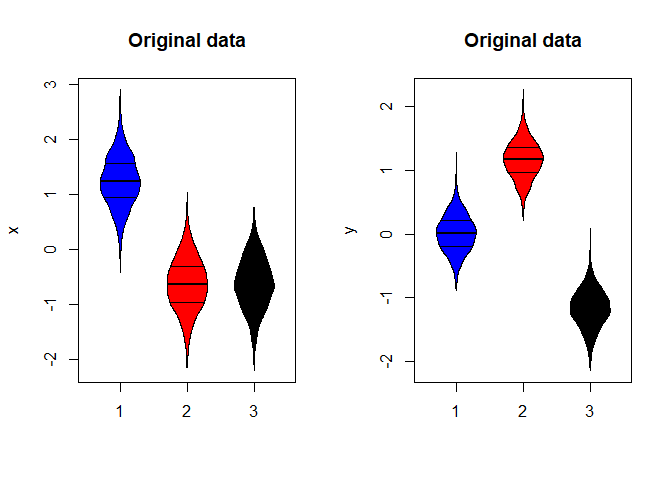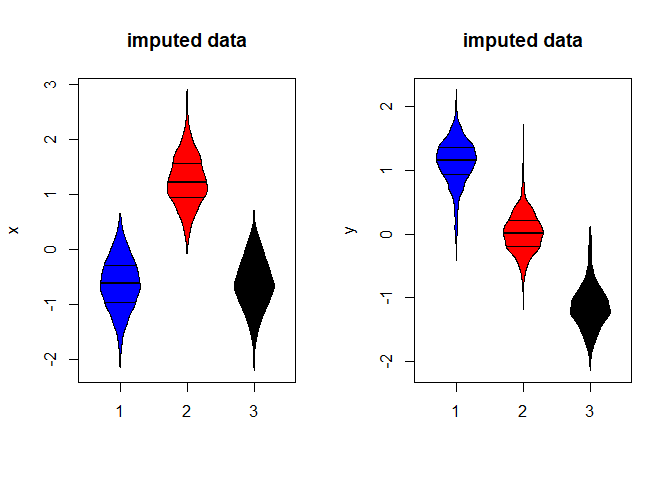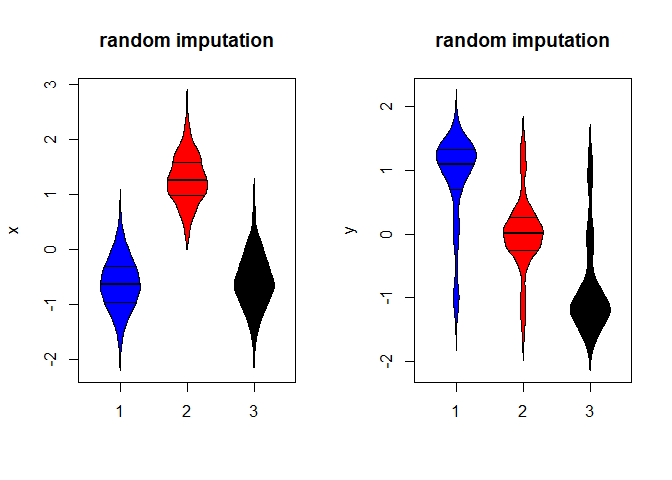In particular for y the distribution by cluster is quite far away from the original distribution for the random imputation based clustering.

External validation: rand index

Below we compare the rand index between true and fitted cluster assignment. For all cases we obtain

It is considerably lower for random imputation:

Of course, it is much higher on the (small number of) complete cases.

The function also computes a variety of other stats

Variance reduction

We assess quality of clusters, we compute the sum of squares within each cluster, sum up this value and compare it by the total sum of squares.

We se a reduction of about 27% using only 3 clusters, most strikingly for x and y because that these variables define the subspace of the true clusters.

More clusters will capture the random distribution of the other variables

We’ll do this exercise for a several values of nr_cluster (and need a helper function for that since X is an argument of ClustImpute)

..and put the variances by cluster in a table

While there is a rather gradual improvement for the other variables, x and y have a minimum showing optimality for these variables. Such a plot clearly indicates that 3 clusters are a good choice for this data set.